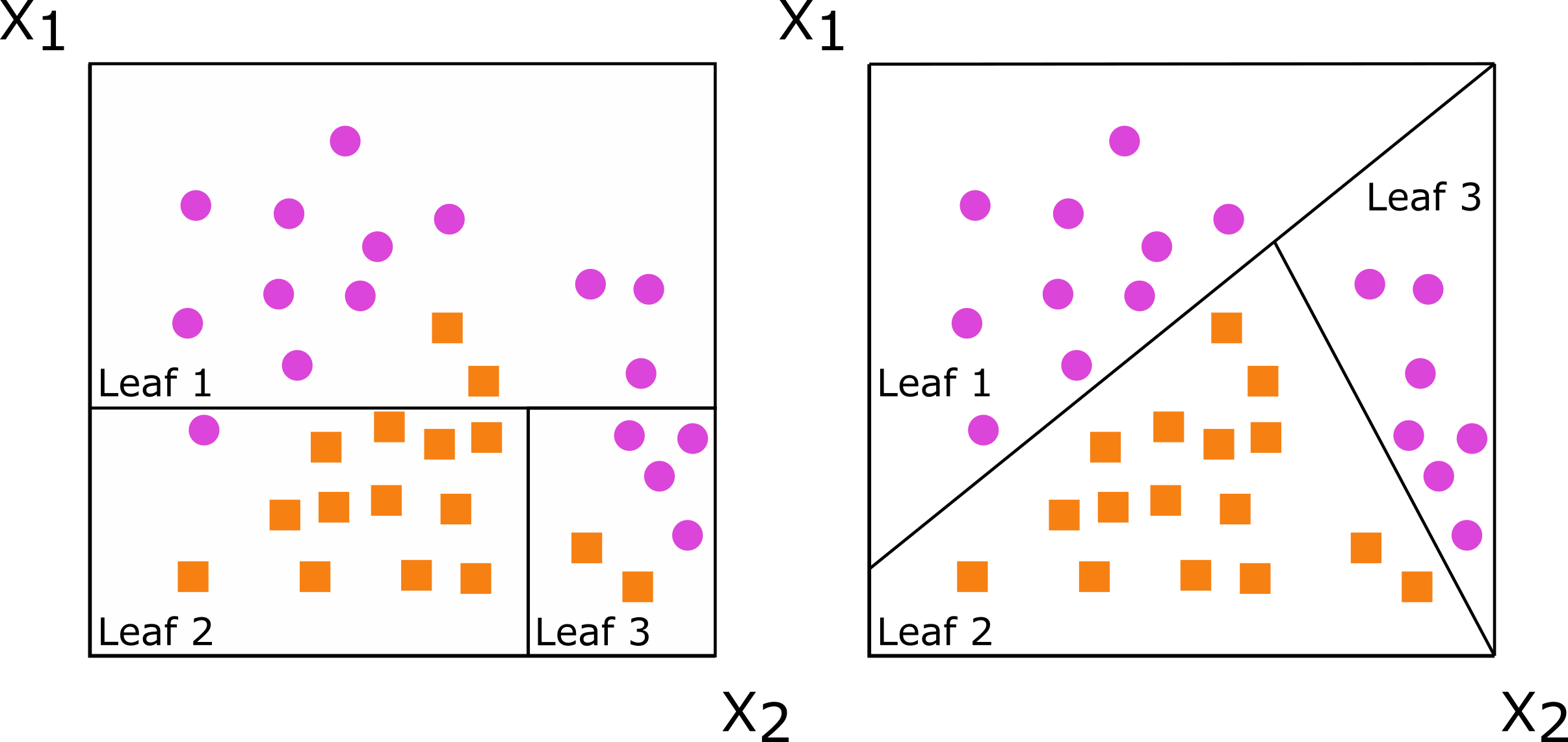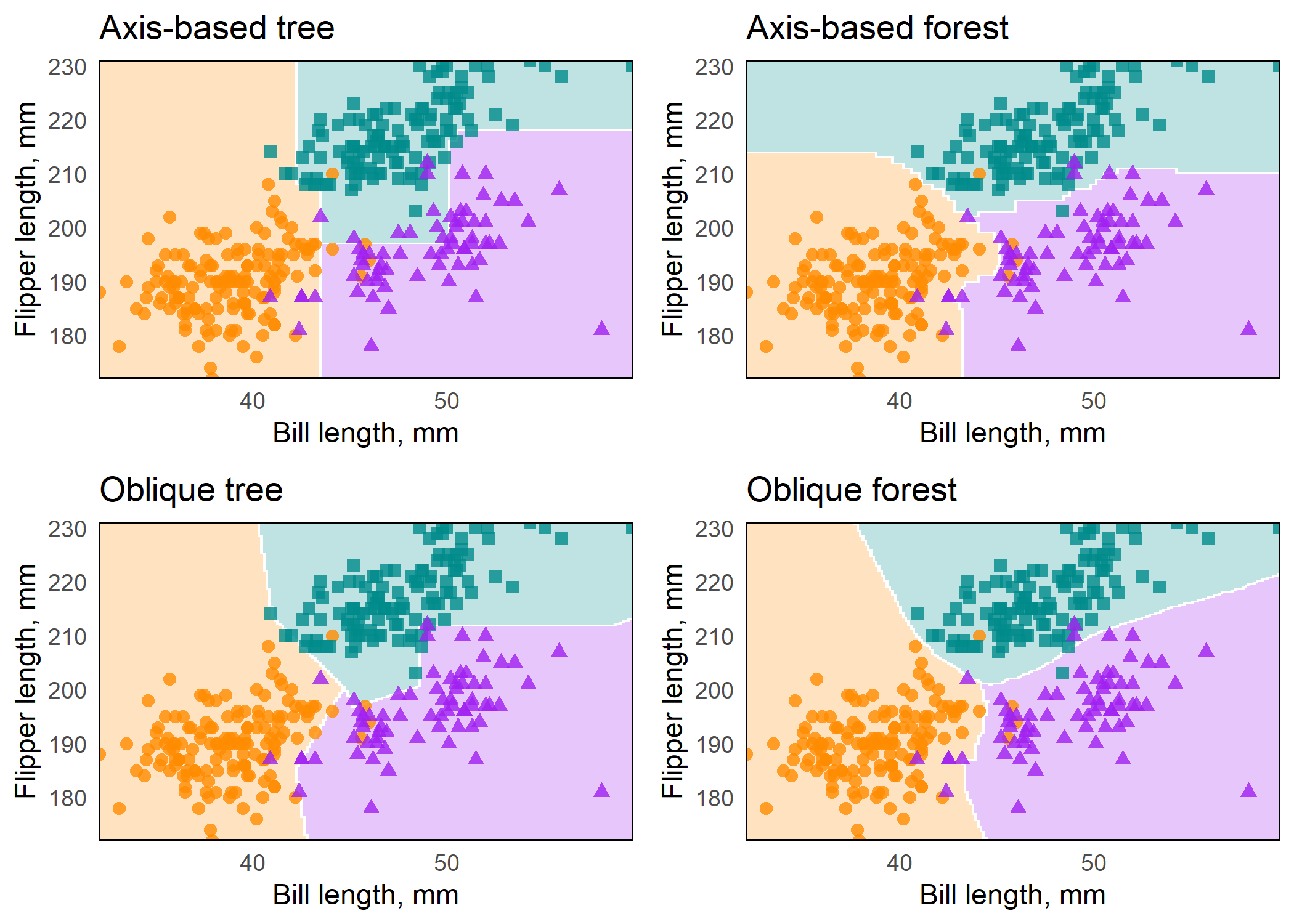Fit, interpret, and make predictions with oblique random forests (RFs).

## Why aorsf?

• Hundreds of times faster than other software for oblique RFs.1

• Accurate predictions.2

• Computes partial dependence and variable importance.2

• Intuitive API with formula based interface.

• Extensive input checks and informative error messages.

## Installation

You can install `aorsf` from CRAN using

``install.packages("aorsf")``

You can install the development version of aorsf from GitHub with:

``````# install.packages("remotes")
remotes::install_github("ropensci/aorsf")``````

## What is an oblique decision tree?

Decision trees are grown by splitting a set of training data into non-overlapping subsets, with the goal of having more similarity within the new subsets than between them. When subsets are created with a single predictor, the decision tree is axis-based because the subset boundaries are perpendicular to the axis of the predictor. When linear combinations (i.e., a weighted sum) of variables are used instead of a single variable, the tree is oblique because the boundaries are neither parallel nor perpendicular to the axis.

Figure: Decision trees for classification with axis-based splitting (left) and oblique splitting (right). Cases are orange squares; controls are purple circles. Both trees partition the predictor space defined by variables X1 and X2, but the oblique splits do a better job of separating the two classes.So, how does this difference translate to real data, and how does it impact random forests comprising hundreds of axis-based or oblique trees? We will demonstrate this using the `penguin` data from the magnificent `palmerpenguins` R package.

``````library(aorsf)
library(tidyverse)

penguins_orsf <- penguins_orsf %>%
mutate(bill_length_mm = as.numeric(bill_length_mm),
flipper_length_mm = as.numeric(flipper_length_mm))``````

We will also use this function to make several plots:

``````plot_decision_surface <- function(predictions, title, grid){

# this is not a general function for plotting
# decision surfaces. It just helps to minimize
# copying and pasting of code.

colnames(predictions) <- levels(penguins_orsf\$species)

class_preds <- bind_cols(grid, predictions) %>%
Chinstrap,
Gentoo)) %>%
group_by(flipper_length_mm, bill_length_mm) %>%
arrange(desc(value)) %>%
slice(1)

cols <- c("darkorange", "purple", "cyan4")

ggplot(class_preds, aes(bill_length_mm, flipper_length_mm)) +
geom_contour_filled(aes(z = value, fill = name),
alpha = .25) +
geom_point(data = penguins_orsf,
aes(color = species, shape = species),
size = 2,
alpha = 0.8) +
scale_color_manual(values = cols) +
scale_fill_manual(values = cols) +
labs(x = "Bill length, mm",
y = "Flipper length, mm") +
theme_minimal() +
scale_x_continuous(expand = c(0,0)) +
scale_y_continuous(expand = c(0,0)) +
theme(panel.grid = element_blank(),
panel.border = element_rect(fill = NA),
legend.position = '') +
labs(title = title)

}``````

We also use a grid of points for plotting decision surfaces:

``````grid <- expand_grid(

flipper_length_mm = seq(min(penguins_orsf\$flipper_length_mm),
max(penguins_orsf\$flipper_length_mm),
len = 200),
bill_length_mm = seq(min(penguins_orsf\$bill_length_mm),
max(penguins_orsf\$bill_length_mm),
len = 200)
)``````

We use `orsf` with `mtry=1` to fit axis-based trees and random forests. Then we use `orsf_update` to expand axis-based trees to oblique ones, and single trees to forests:

``````fit_axis_tree <- penguins_orsf %>%
orsf(species ~ bill_length_mm + flipper_length_mm,
n_tree = 1,
mtry = 1,
tree_seeds = 106760)

fit_axis_forest <- fit_axis_tree %>%
orsf_update(n_tree = 500)

fit_oblique_tree <- fit_axis_tree %>%
orsf_update(mtry = 2)

fit_oblique_forest <- fit_oblique_tree %>%
orsf_update(n_tree = 500)

preds <- list(fit_axis_tree,
fit_axis_forest,
fit_oblique_tree,
fit_oblique_forest) %>%
map(predict, new_data = grid, pred_type = 'prob')

titles <- c("Axis-based tree",
"Axis-based forest",
"Oblique tree",
"Oblique forest")

plots <- map2(preds, titles,
.f = plot_decision_surface,
grid = grid)``````

Figure: Axis-based and oblique decision surfaces from a single tree and an ensemble of 500 trees. Axis-based trees have boundaries perpendicular to predictor axes, whereas oblique trees can have boundaries that are neither parallel nor perpendicular to predictor axes. Axis-based forests tend to have ‘step-function’ decision boundaries, while oblique forests tend to have smooth decision boundaries.## Examples

`orsf()` fits several types of oblique RFs. My personal favorite is the accelerated oblique survival RF because it has a great combination of prediction accuracy and computational efficiency (see JCGS paper).2

``````
library(aorsf)

set.seed(329730)

index_train <- sample(nrow(pbc_orsf), 150)

pbc_orsf_train <- pbc_orsf[index_train, ]
pbc_orsf_test <- pbc_orsf[-index_train, ]

fit <- orsf(data = pbc_orsf_train,
formula = Surv(time, status) ~ . - id,
oobag_pred_horizon = 365.25 * 5)``````

### Inspect

Printing the output from `orsf()` will give some information and descriptive statistics about the ensemble.

``````fit
#> ---------- Oblique random survival forest
#>
#>      Linear combinations: Accelerated Cox regression
#>           N observations: 150
#>                 N events: 52
#>                  N trees: 500
#>       N predictors total: 17
#>    N predictors per node: 5
#>  Average leaves per tree: 10.238
#> Min observations in leaf: 5
#>       Min events in leaf: 1
#>           OOB stat value: 0.83
#>            OOB stat type: Harrell's C-index
#>      Variable importance: anova
#>
#> -----------------------------------------``````
• See print.ObliqueForest for a description of each line in the printed output.

• See orsf examples for more details on controlling ORSF ensemble fits and using them in prediction modeling workflows.

### Variable importance

The importance of individual variables can be estimated in three ways using `aorsf`:

• negation2: Each variable is assessed separately by multiplying the variable’s coefficients by -1 and then determining how much the model’s performance changes. The worse the model’s performance after negating coefficients for a given variable, the more important the variable. This technique is promising b/c it does not require permutation and it emphasizes variables with larger coefficients in linear combinations, but it is also relatively new and hasn’t been studied as much as permutation importance. See Jaeger, (2023) for more details on this technique.

``````orsf_vi_negate(fit)
#>         bili          sex       copper        stage          age          ast
#>  0.117180683  0.058528338  0.033761789  0.026655509  0.022144911  0.019139095
#>      protime       hepato        edema      ascites      albumin         chol
#>  0.016879701  0.011605852  0.010634489  0.009580159  0.008336260  0.007633992
#>          trt      spiders     alk.phos         trig     platelet
#>  0.002705027  0.002662017  0.002413369  0.001197399 -0.003386483``````
• permutation: Each variable is assessed separately by randomly permuting the variable’s values and then determining how much the model’s performance changes. The worse the model’s performance after permuting the values of a given variable, the more important the variable. This technique is flexible, intuitive, and frequently used. It also has several known limitations

``````orsf_vi_permute(fit)
#>         bili       copper          age        stage          sex          ast
#>  0.050536719  0.016394807  0.013793348  0.013204760  0.010261860  0.010101841
#>        edema      ascites      protime      albumin       hepato         chol
#>  0.008298456  0.008148291  0.007630773  0.006667768  0.006141770  0.002881687
#>      spiders         trig     alk.phos     platelet          trt
#>  0.001669604  0.001047642 -0.000301684 -0.001417230 -0.001665785``````
• analysis of variance (ANOVA)3: A p-value is computed for each coefficient in each linear combination of variables in each decision tree. Importance for an individual predictor variable is the proportion of times a p-value for its coefficient is < 0.01. This technique is very efficient computationally, but may not be as effective as permutation or negation in terms of selecting signal over noise variables. See Menze, 2011 for more details on this technique.

``````orsf_vi_anova(fit)
#>         bili       copper          age        stage          sex          ast
#>  0.050536719  0.016394807  0.013793348  0.013204760  0.010261860  0.010101841
#>        edema      ascites      protime      albumin       hepato         chol
#>  0.008298456  0.008148291  0.007630773  0.006667768  0.006141770  0.002881687
#>      spiders         trig     alk.phos     platelet          trt
#>  0.001669604  0.001047642 -0.000301684 -0.001417230 -0.001665785``````

You can supply your own R function to estimate out-of-bag error when using negation or permutation importance (see oob vignette)

### Partial dependence (PD)

Partial dependence (PD) shows the expected prediction from a model as a function of a single predictor or multiple predictors. The expectation is marginalized over the values of all other predictors, giving something like a multivariable adjusted estimate of the model’s prediction.

The summary function, `orsf_summarize_uni()`, computes PD for as many variables as you ask it to, using sensible values.

``````orsf_summarize_uni(fit, n_variables = 2)
#>
#> -- bili (VI Rank: 1) ----------------------------
#>
#>         |---------------- Risk ----------------|
#>   Value      Mean    Median     25th %    75th %
#>  <char>     <num>     <num>      <num>     <num>
#>    0.70 0.2043124 0.1288782 0.05502854 0.3130744
#>    1.30 0.2193531 0.1430383 0.06680735 0.3352729
#>    3.20 0.2843241 0.2228119 0.12421850 0.4304313
#>
#> -- copper (VI Rank: 2) --------------------------
#>
#>         |---------------- Risk ----------------|
#>   Value      Mean    Median     25th %    75th %
#>  <char>     <num>     <num>      <num>     <num>
#>    39.0 0.2308500 0.1358346 0.05536305 0.3575617
#>    68.0 0.2415171 0.1482876 0.06189812 0.3682164
#>     111 0.2727120 0.1846062 0.08723814 0.4084767
#>
#>  Predicted risk at time t = 1826.25 for top 2 predictors``````

For more on PD, see the vignette

### Individual conditional expectations (ICE)

Unlike partial dependence, which shows the expected prediction as a function of one or multiple predictors, individual conditional expectations (ICE) show the prediction for an individual observation as a function of a predictor.

For more on ICE, see the vignette

## Comparison to existing software

Comparisons between `aorsf` and existing software are presented in our JCGS paper. The paper:

• describes `aorsf` in detail with a summary of the procedures used in the tree fitting algorithm

• runs a general benchmark comparing `aorsf` with `obliqueRSF` and several other learners

• reports prediction accuracy and computational efficiency of all learners.

• runs a simulation study comparing variable importance techniques with oblique survival RFs, axis based survival RFs, and boosted trees.

• reports the probability that each variable importance technique will rank a relevant variable with higher importance than an irrelevant variable.

A more hands-on comparison of `aorsf` and other R packages is provided in orsf examples

1. Jaeger BC, Long DL, Long DM, Sims M, Szychowski JM, Min YI, Mcclure LA, Howard G, Simon N. Oblique random survival forests. Annals of applied statistics 2019 Sep; 13(3):1847-83. DOI: 10.1214/19-AOAS1261

2. Jaeger BC, Welden S, Lenoir K, Speiser JL, Segar MW, Pandey A, Pajewski NM. Accelerated and interpretable oblique random survival forests. Journal of Computational and Graphical Statistics Published online 08 Aug 2023. DOI: 10.1080/10618600.2023.2231048

3. Menze BH, Kelm BM, Splitthoff DN, Koethe U, Hamprecht FA. On oblique random forests. Joint European Conference on Machine Learning and Knowledge Discovery in Databases 2011 Sep 4; pp. 453-469. DOI: 10.1007/978-3-642-23783-6_29